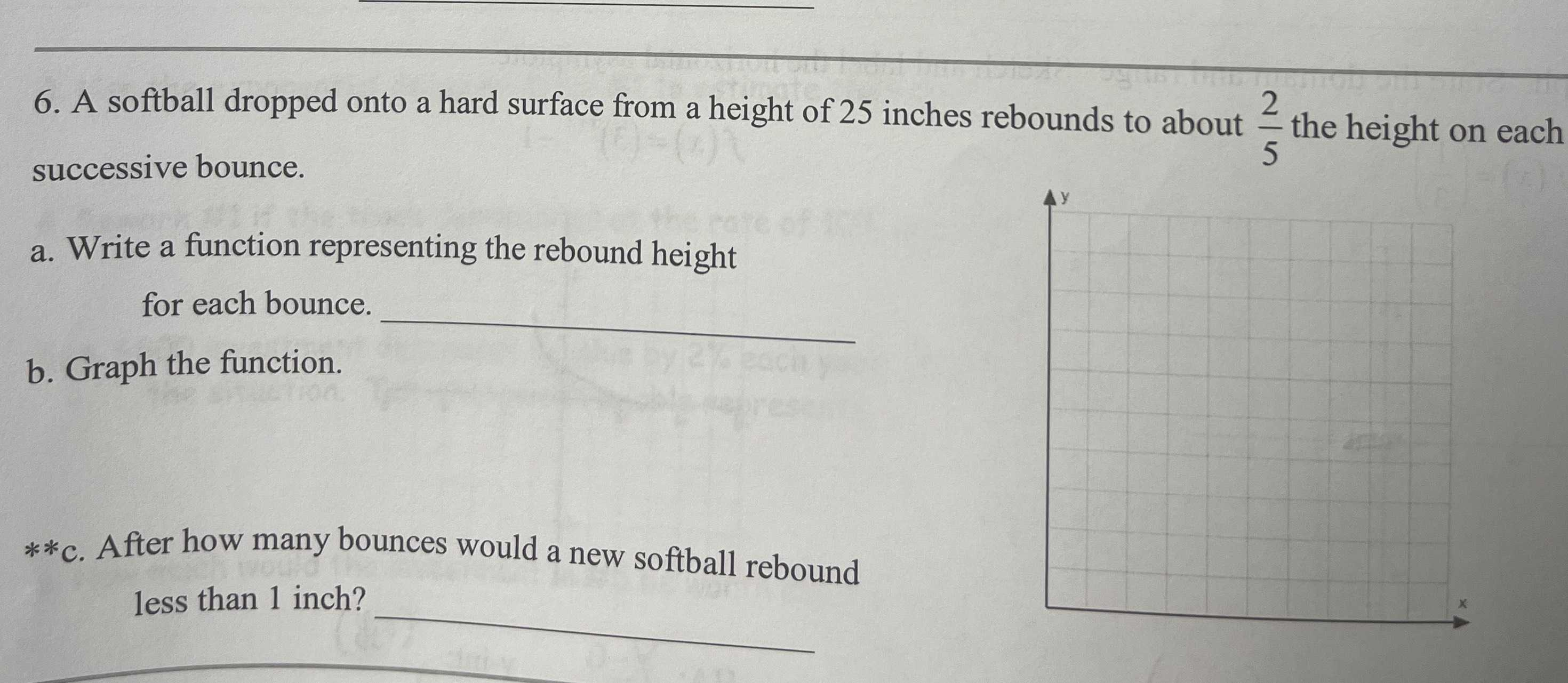### ¿Todavía tienes preguntas de matemáticas?

Pregunte a nuestros tutores expertos
Algebra
Pregunta6. A softball dropped onto a hard surface from a height of $$25$$ inches rebounds to about $$\frac { 2 } { 5 }$$ the height on each successive bounce. a. Write a function representing the rebound height for each bounce. Graph the function. **c. After how many bounces would a new softball rebound less than $$1$$ inch?

$$h(n)= 25(\frac{2}{5})^{n}$$